We are thrilled to have a guest post in our blog by Dr. Hershel Safer. Dr.Safer is an expert in taking the most advanced mathematics, statistics and machine learning techniques and generating the most robust credit risk models possible. Through many years of experience in research and development of models, Dr.Safer has developed a set of guidelines which prove essential when developing new credit risk models. In this guest blog post, we’d like to share his cookbook for the development of some of the most basic functions used in modelling: Weight of Evidence (WOE), Information Value (IV) and PSI (Population Stability Index).

## Introduction

In credit risk modelling, as in other fields where a predictive model is built based on raw historical data, preparing the data for the training is the most crucial stage in the creation of a strong model. The statistical nature of many raw features is often not perfectly aligned with the requirements of various training algorithms and may result in inferior models. Thus, preparing the data properly will yield stronger results.

In credit risk, as in other areas of financial and behavioral modelling, certain scenarios occur repeatedly. Applying the appropriate data transformations can yield vastly improved results. This post explores several such functions along with the relevant mathematical and statistical details. The post can provide a solid foundation for understanding, implementing, and using these functions in your modelling projects.

In this post, the term “characteristic” means “variable” or “feature.” An “attribute” is a specific value taken by a characteristic. This terminology is not universal in machine learning, but it is common in the credit risk literature.

## Weight of Evidence (WOE)

Weight of Evidence (WOE) is used to assess the predictive value of individual attribute values of a characteristic.

Suppose that the sample has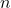negative instances and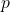positives, withandbeing the numbers of negative and positive instances with attribute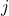. A common way to represent the data for a characteristic with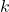attributes is atable. Each row corresponds to an attribute and each column to a value of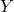(0 or 1). Each cell contains the number of observations with the corresponding attribute and target values.

The WOE for attributeis. This can be rewritten as; this highlights WOE as being the difference between the log odds of the attribute and the population log odds. Attributes with log odds close to that of the population have little WOE.

The Weight of Evidence (WOE) transformation replaces each attribute with a risk value. When, the probability of observingfor instances with attributeis above average for the sample, and vice versa for. WOE also standardizes each characteristic, so the parameters in logistic regression can be directly compared.

#### Implementation notes:

• Bin continuous values so that each attribute has approximately the same number of observations. Alternatively, use a decision tree to select the bins borders for each continuous variable.
• Put missing values in a separate row and treat them as another attribute.
• WOE is undefined for any row that has a zero in a cell. Change the 0 counts to 1; this small change to the data allows WOE to be calculated for all rows. An alternative is to add 0.5 to every bin.
• Feature values with similar weight of evidence are sometimes merged (coarse classing). For continuous or other ordered variables, only adjacent classes should be combined.
• Theandvalues are fractions within the corresponding column.

Logistic regression tries to predict the conditional logit or conditional log-odds of. The conditional logit can be written as the sum of the sample log-odds and the log-density ratio; the latter is the WOE.Since the sample log-odds is constant, logistic regression effectively tries to predict the WOE.

The naive Bayes model can be writtenSo the conditional logit equals the sum of the individual WOE vectors.

A semi-naive model relaxes the assumption that the predictors are independent:The individual WOE vectors are estimated separately, and thecoefficients are scalars.

## Information Value

WOE describes the relationship between an attribute value and a binary target variable; the Information Value (IV) measures the predictive power of a characteristic, i.e., to what extent it can be used to separate observations withfrom those with. IV is a weighted sum of the WOE values:

WOE considers only the relative risk of each bin, without regard to the proportion of observations in the bin. The terms used to compute Information Value can be used to assess the relative contribution of each bin.

IV is always non-negative, and higher values indicate that the value is more informative. Features with information value less than 0.02 are not useful for prediction, 0.02–0.1 are weakly predictive, 0.3–0.5 are highly predictive, and greater than 0.5 are too good to be true. That said, features that are weak on their own may be useful in combination with other features. IV is sensitive to the binning of continuous values and to the total number of groups. It does not have an associated statistical test, so variables are often selected based on the information value or the chi-squared test, but the Gini coefficient is used for the scorecard.

#### Population Stability Index

The Population Stability Index (PSI) measures a shift in distributions of a measure across population groups, similar to the chi-squared statistic. A common use in credit risk is to measure the drift between two times. The formulation is the same as for IV, but withandreferring to the new observation, andandreferring to the expected values (previous time or development sample).

A larger PSI indicates a larger shift in the distribution compared to the benchmark, but does not say anything about the direction of the shift. Values less than 0.1 indicate little drift, between 0.1 and 0.25 indicates moderate drift (cause for concern), and greater than 0.25 indicates large drift (possible problems).WOE considers only the relative risk of each bin, without regard to the proportion of observations in the bin. The terms used to compute Information Value can be used to assess the relative contribution of each bin.

IV is always non-negative, and higher values indicate that the value is more informative. Features with information value less than 0.02 are not useful for prediction, 0.02–0.1 are weakly predictive, 0.3–0.5 are highly predictive, and greater than 0.5 are too good to be true. That said, features that are weak on their own may be useful in combination with other features. IV is sensitive to the binning of continuous values and to the total number of groups. It does not have an associated statistical test, so variables are often selected based on the information value or the chi-squared test, but the Gini coefficient is used for the scorecard.

#### Population Stability Index

The Population Stability Index (PSI) measures a shift in distributions of a measure across population groups, similar to the chi-squared statistic. A common use in credit risk is to measure the drift between two times. The formulation is the same as for IV, but withandreferring to the new observation, andandreferring to the expected values (previous time or development sample).

A larger PSI indicates a larger shift in the distribution compared to the benchmark, but does not say anything about the direction of the shift. Values less than 0.1 indicate little drift, between 0.1 and 0.25 indicates moderate drift (cause for concern), and greater than 0.25 indicates large drift (possible problems).

[/fusion_text][/fusion_builder_column][/fusion_builder_row][/fusion_builder_container]